Common Factors (and friends) MathBitsNotebook.com Terms of Use   Contact Person: Donna RobertsWhen two (or more) integers are multiplied together, the answer is called a product.
The integers that were multiplied together are called the factors of the product.

 3 • 6 = 18 (3 and 6 are factors of 18)A number can have a list of factors. Factors of 18 are 1, 2, 3, 6, 9, and 18.

When considering the lists of factors of two (or more) integers, a common factor
is factor that is shared by (found in) both (or all) of the lists.

One method of finding common factors is by "listing" the factors of each number
and seeing what factors they have in "common" (they share).

 Consider the factors of 18 and 24. Factors of 18: 1, 2, 3, 6, 9, 18 Factors of 24: 1, 2, 3, 4, 6, 8, 12, 24 The common factors are 1, 2, 3, 6. (1, 2, 3 and 6 appear in both lists of factors)

The greatest common factor (GCF) of two (or more) integers is the largest integer
that divides exactly into both (or all) numbers.

It is the largest integer that is a factor of both (or all) numbers.
It is the largest of the common factors.

Note: GCF is sometimes referred to as HCF (highest common factor).

 Consider the numbers 18, 24, and 36. Their factors are: Factors of 18: 1, 2, 3, 6, 9, 18 Factors of 24: 1, 2, 3, 4, 6, 8, 12, 24 Factors of 36: 1, 2, 3, 4, 6, 9, 12, 18, 36 Their common factors are 1, 2, 3, and 6. Their greatest common factor is 6. (6 is the largest integer that will divide evenly into all three numbers)

Another method of finding the greatest common factor is by using prime factorization.
This method is particularly useful when the numbers are LARGE
and "listing" the factors becomes too time consuming.

What is the greatest common factor of 4080 and 1920?
Listing the factors of these numbers would be too tedious.
Instead, let's find the prime factorizations of these numbers.
 4080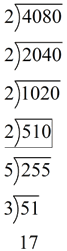1920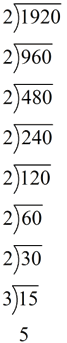4080 = 2 x 2 x 2 x 2 x 5 x 3 x 17 = 24 x 5 x 3 x 17 1920 = 2 x 2 x 2 x 2 x 2 x 2 x 2 x 3 x 5 = 27 x 3 x 5 Shared (in common): 2 x 2 x 2 x 2 x 3 x 5 = 240 The greatest common factor is 240.Common factors find their way into all types of problems. We will be looking at common factors and their connection to the distributive property, a concept that will be used frequently in algebra. Consider the following examples: 42 + 35 = 7(6 + 5) where 7 is the GCF of 42 and 35. 36 + 81 = 9(4 + 9) where 9 is the GCF of 36 and 81. 75 + 100 = 25(3 + 4) where 25 is the GCF of 75 and 100. In these examples, the distributive property is used to express a sum of two positive integers with a common factor, as a multiple of a sum of two positive integers with no common factor.See Example 4 under Factoring - Numerical Examples
for more on this topic.

Common factors are even being used when you are reducing or simplifying fractions.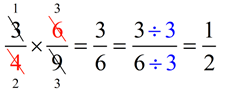The common factor of 3 and 9 (which is 3) was used to reduce a portion of the problem. The common factor of 4 and 6 (which is 2) was used to reduce another portion of the problem.
The common factor of 3 and 6 (which is 3) was used to arrive at the simplest form of the answer.The
least common multiple (LCM) is the smallest number into which
two (or more) integers will divide exactly.

It is the smallest number containing all factors of both numbers.

When looking for a LCM, list the multiples of each of the numbers.
(That is, multiply the number
x2, x3, x4, x5, ...)
Keep the lists going for both numbers until a common (shared) number from each list appears.

 Least Common Multiple of 3 and 7 Multiples of 3: 3, 6, 9, 12, 15, 18, 21, ... Multiples of 7: 7, 14, 21, ... The least common multiple is 21. (21 is the smallest number into which 3 and 7 divide exactly)

There are a lot of common multiples, but only one least common multiple:
Multiples of 3: 3, 6, 9, 12, 15, 18, 21, 24, 27 30 33, 36, 39, 42, 45, ...
Multiples of 7: 7, 14, 21, 28, 35, 42, 29, ...
Notice that 42 is also a common multiple of 3 and 7 (and there are many others).
But the "least" (the smallest) common multiple is 21.

The least common multiple is being used when finding a common denominator for working with fractions. When adding the fractions in the problem below, the least common multiple of 6 and 4 is 12, making 12 the least common denominator.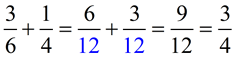The common factor of 3 (for 9 and 12) was used to reduce the fraction to its simplest form.

As we saw in the "greatest common factor" example above, "listing" works nicely as long as the numbers are relatively small. The same is true for finding the "least common multiple".
In the previous example, we used
prime factorization to obtain:

4080 = 2 x 2 x 2 x 2 x 5 x 3 x 17 = 24 x 5 x 3 x 17

1920 = 2 x 2 x 2 x 2 x 2 x 2 x 2 x 3 x 5 = 27 x 3 x 5

Now, the least common multiple will contain all factors of both numbers.
The
LCM is 27 x 3 x 5 x 17 = 32,640
(The factor of 24 is already covered within 27.)The GCF and LCM can be observed using Prime Factor Diagrams.
These are Venn Diagrams containing prime factors.

Find the GCF and LCM for 24 and 30.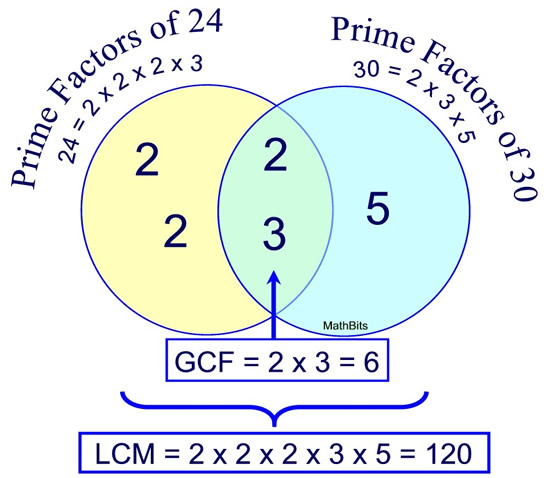The GCF is the product of the prime factors that overlap.
The LCM is the product of all of the prime factors observed in the diagram.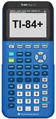For help with LCM and GCF on your calculator, click here.